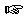### Section 11

Problem 1:
Develop a function that consumes two numbers and subtracts the second number from the first without using the minus operator.
Warning: A student may use "binary" recursion rather than natural recursion on just the summand. Be prepared to grade harshly or to ask for detailed explanations.Solution

Problem 2:
Develop the function mod-list. The function consumes two natural numbers and produces a list of numbers between zero and the first number that are not evenly divisible by the second number. Your function should produce an error if the second number is zero.Solution

Problem 3:

One way to describe motion is to compute the location of an object at a given point in time:

``````
; Nat -> Posn
; compute location of an object at time t if is at START and moves at VELOCITY
(define (location t)
(add START (mul t VELOCITY)))

(define START ...)
(define VELOCITY ...)
``````
Assuming the object is at START and moves at VELOCITY then we multiply VELOCITY with t and add START to find out where the object is at time t.

Develop the function diag2. The function consumes a time t (natural number) and computes the movement of the object as a list of point. The first point describes where the object was at time t, the last one describes it for time 1.Solution

Problem 4:
Develop the function bm. The function consumes two numbers (`n` and `m`) and a symbol (`s`). It produces a list of `n` lists. Each of these lists is a list of `m` symbols, namely, `s`.
Hint: You need an auxiliary function.

Consider the following modification. Instead of using the same symbol over and over again, create a string that says "cell23" where 2 and 3 are (running) indices. Recall that `string-append` concatenates two strings and that `number->string` creates a string representation from a number. This variant is, for example, useful in Web programming.Solution

Problem 5:

The following program fragment draws and clears a balloon on a canvas at time `n`:

``````
; Nat -> true
(define (clear-balloon n)
(and (clear-solid-disk (make-posn 100 (* n 5)) RADIUS 'red)
(clear-solid-line (make-posn 100 (+ (* n 5) RADIUS))
(make-posn 100 (+ (* n 5) RADIUS LENGTH))
'black)))

; Nat -> true
(define (draw-balloon n)
(and (draw-solid-disk (make-posn 100 (* n 5)) RADIUS 'red)
(draw-solid-line (make-posn 100 (+ (* n 5) RADIUS))
(make-posn 100 (+ (* n 5) RADIUS LENGTH))
'black)))

Design the function `balloons`. It consumes a natural number. Its task is to clear and draw a balloon that many times, at times `n` through `1`. Its result is `true.` To achieve a realistic effect, the function should sleep for a while (see docs of draw.ss teachpack) after drawing a balloon.Solution